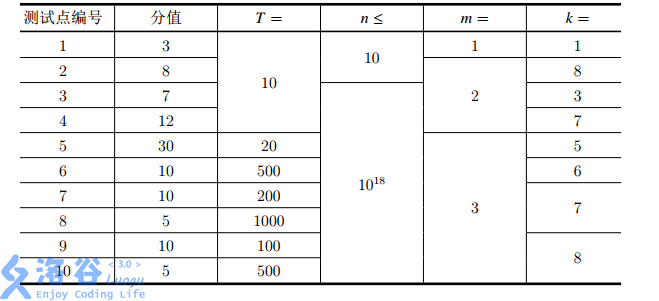P4007 小 Y 和恐怖的奴隶主

• 171通过
• 556提交
• 题目提供者 洛谷
• 评测方式 云端评测
• 标签 期望 概率论,统计 矩阵加速,矩阵优化 清华集训 2017 O2优化 高性能
• 难度 NOI/NOI+/CTSC
• 时空限制 2000ms / 512MB
• 提示：收藏到任务计划后，可在首页查看。

题目背景

“A fight? Count me in!” 要打架了，算我一个。

“Everyone, get in here!” 所有人，都过来！

题目描述

小 Y 是一个喜欢玩游戏的 OIer。一天，她正在玩一款游戏，要打一个 Boss。

虽然这个 Boss 有 $10^{100}$ 点生命值，但它只带了一个随从——一个只有 $m$ 点生命值的“恐怖的奴隶主”。

这个“恐怖的奴隶主”有一个特殊的技能：每当它被扣减生命值但没有死亡（死亡即生命值 $\leq 0$），且 Boss 的随从数量小于上限 $k$，便会召唤一个新的具有 $m$ 点生命值的“恐怖的奴隶主”。

现在小 Y 可以进行 $n$ 次攻击，每次攻击时，会从 Boss 以及 Boss 的所有随从中的等概率随机选择一个，并扣减 $1$ 点生命值，她想知道进行 $n$ 次攻击后扣减 Boss 的生命值点数的期望。为了避免精度误差，你的答案需要对 $998244353$ 取模。

输入输出格式

输入格式：

输入第一行包含三个正整数 $T, m, k$，$T$ 表示询问组数，$m, k$ 的含义见题目描述。

接下来 $T$ 行，每行包含一个正整数 $n$，表示询问进行 $n$ 次攻击后扣减Boss的生命值点数的期望。

输出格式：

输出共 $T$ 行，对于每个询问输出一行一个非负整数，表示该询问的答案对 $998244353$ 取模的结果。

可以证明，所求期望一定是一个有理数，设其为 $p / q$ $(\mathrm{gcd}(p,q) = 1)$，那么你输出的数 $x$ 要满足 $p \equiv qx \pmod{998244353}$。

输入输出样例

输入样例#1： 复制
3 2 6
1
2
3
输出样例#1： 复制
499122177
415935148
471393168

说明

【样例 $1$ 解释】

对于第一次询问，第一次攻击有 $\frac{1}{2}$ 的概率扣减 Boss 的生命值，有 $\frac{1}{2}$ 的概率扣减随从的生命值，所以答案为 $\frac{1}{2}$。$1 \equiv 2 \times 499122177 \pmod{998244353}$。

对于第二次询问，如果第一次攻击扣减了 Boss 的生命值，那么有 $\frac{1}{2}$ 的概率第二次攻击仍扣减 Boss 的生命值，有 $\frac{1}{2}$ 的概率第二次攻击扣减随从的生命值；如果第一次攻击扣减了随从的生命值，那么现在又新召唤了一个随从（“恐怖的奴隶主”），于是有 $\frac{1}{3}$ 的概率第二次攻击扣减 Boss 的生命值，有 $\frac{2}{3}$ 的概率第二次攻击扣减随从的生命值。所以答案为 $\frac{1}{2}\times\frac{1}{2}\times2+\frac{1}{2}\times\frac{1}{2}\times1+\frac{1}{2}\times\frac{1}{3}\times1+\frac{1}{2}\times\frac{2}{3}\times0 = \frac{11}{12}$。 $11 \equiv 12 \times 415935148\pmod{998244353}$。

【提示】

题目顺序可能与难度无关。

【子任务】

在所有测试点中，$1 \leq T \leq 1000, 1 \leq n \leq {10}^{18}, 1 \leq m \leq 3, 1 \leq k \leq 8$。

各个测试点的分值和数据范围如下：提示
标程仅供做题后或实在无思路时参考。
请自觉、自律地使用该功能并请对自己的学习负责。
如果发现恶意抄袭标程，将按照I类违反进行处理。